Law of Corresponding States is also known as principle of corresponding states or theorem of corresponding states. As per this law, all liquids nearly have similar compressibility factor. And due to this, all such perfect gasses deviate from their ideal behavior, which is approximately to the same degree.

But there is a specific condition when this law is applicable. The outcome can be witnessed when these gasses are in their reduced pressure and reduced temperature state.

Other Definition

Law of Corresponding States can also be stated as an empirical law. As per this law, it summarizes the findings for many real gasses. These findings are based on the equations which describe the similarity of ideal gasses.

Factors which reflects the similarity of these gasses are,

• Volume
• Pressure
• Reduced temperature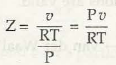Pu = ZRT

Consider the figure next: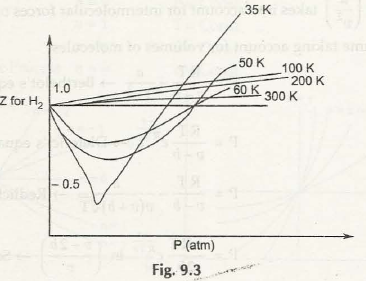All these terms are based on the associationwith critical point.

The definition of reduced variables is mainly based on critical variables.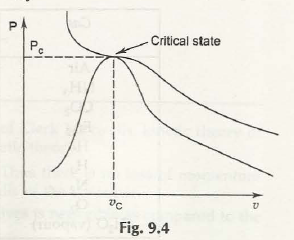Mathematically Z = f(P r, T r)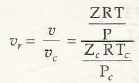For the equation above consider this figure: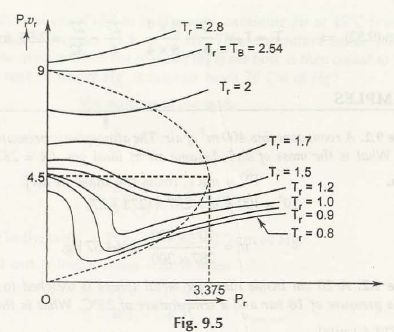Links of Next Mechanical Engineering Topics:-### Customer Reviews

My Homework Help
Rated 5.0 out of 5 based on 510 customer reviews at